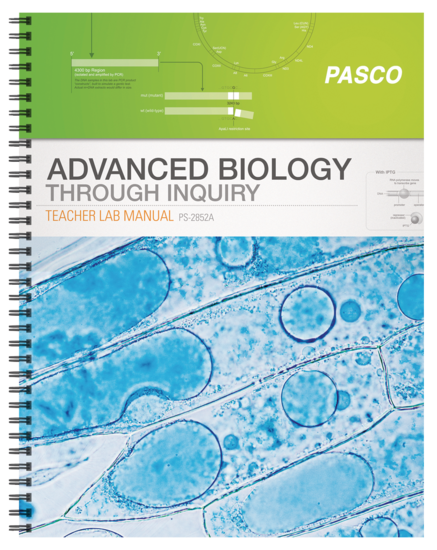# Mathematical Modeling of Evolution

Students work with a mathematical model and computer simulation to explore how inheritance patterns and gene frequencies change in a population.

Student Files

 17_ABI_Mathematical_Modeling_of_Evolution_S.docx 112.06 KB 17_ABI_Mathematical_Modeling_of_Evolution_S.pdf 247.22 KB 17_ABI_Mathematical_Modeling_Spreadsheet_S.xlsx 881.44 KB

Teacher Files